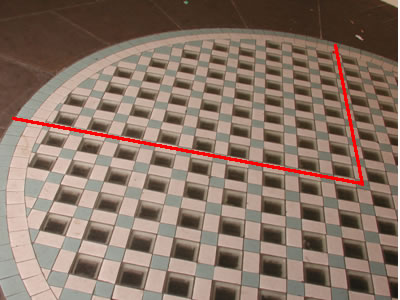You may also likeSquare Pegs

Which is a better fit, a square peg in a round hole or a round peg in a square hole?Floored

A floor is covered by a tessellation of equilateral triangles, each having three equal arcs inside it. What proportion of the area of the tessellation is shaded?Bull's Eye

What fractions of the largest circle are the two shaded regions?

Squaring the Circle

Age 11 to 14 Challenge Level:

Here is a solution sent in by Andrei of School 205, Bucharest. Does anyone think they can do better? Well done Andrei.

I first looked up to find the quarter square in the figure of the problem. From reasons of symmetry, I delimitated it, and the result is in the figure below, where the two perpendicular diameters are in red:This procedure was necessary because the figure does not give the whole circle.

I counted as precise as I could each type of pieces, including parts of pieces on the borders. The results, for the quarter of circle, are in the table below:

Type of piece and location Number of pieces in a quarter circle
Big transparent square, inner part 47
White rectangular pieces, inner part 94.5
Blue-grey squares, inner part 54
White squares, circumference 28
Blue-grey squares, circumference 29

To determine the total number from each type of pieces, I have to multiply all results by 4:

Type of piece and location Number of pieces in a whole circle
Big transparent square, inner part 188
White rectangular pieces, inner part 378
Blue-grey squares, inner part 216
White squares, circumference 112
Blue-grey squares, circumference 116

I think that my estimate is accurate enough, because I tried to count carefully quarter of pieces on the border and to compensate big parts with small ones. My estimate must be more accurate than 5%.

Now I calculate the area of the pavement, and the areas covered by each colour. This way I shall see the accuracy of my previous estimate.

The pavement is a circle, of radius

20 units (inner part) + 2.5 units (circumference) = 22.5 units

The area of the pavement is the area of the circle, of radius 22.5 units, i.e. 1590 sq units..

Now I calculate the area covered by pieces of each colour:

Type of piece and location Unit area (sq units) Total area (sq units)
Big transparent square, inner part 2.25 423
White rectangular pieces, inner part 1.5 567
Blue-grey squares, inner part 1 216
White squares, circumference 1.5625 175
Blue-grey squares, circumference 1.5625 181.25

Now, the total area of the pavement, obtained by adding the numbers from the last column of the table, is 1562.25 sq units. Comparing this with the result 1590 sq units obtained previously, I find an accuracy of around 1.7%, even higher than what I estimated before.